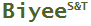Tweet

# Normally Distributed Data Sampling and T-test Simulator

## FREE on-line and off-line tools

(last updated on 2019-11-12)

The tool (on-line version requires IE) simulates the sampling and t-test of two groups of independent, normally distributed data such as the treatment and the control arms of a clinical study. To carry out the simulation, the mean and the standard deviation of each group, which are unknown normally, need to be hypothetically specified. The simulation generates random numbers as samples based on the specified mean and standard deviation. It shows the relative distribution of the sampled data in the charts above and below the button Simulate, then it computes the sample statistics – mean, standard deviation, t value of Student’s t-test, one-tailed and two-tailed confidence levels.

The simulator can help provide some sense about the outcome of a study under different scenarios (e.g. different standard deviations, sampling sizes). It can supplement power analysis. For example, suppose a study on a cholesterol lowering drug is about to be conducted and one measure of the outcome is the cholesterol level change in one month. In addition, it is hypothetically assumed that the mean and the standard deviation are -15 mg/dL and 20 mg/dL respectively for the medication arm. The corresponding parameters for the control arm are 0 mg/dL and 10 mg/dL. Different sample sizes can be entered to see how they affect the statistical significance of the difference between the treatment arm and the control arm. The standard deviations and the means can also be altered to see their effects. The same set of parameters can be run multiple times to see how the effect of the inherent random nature.

Please note: Due to the random nature, every run of simulation generates a different set of results though the distributions of the sampled data are governed by the specified means and standard deviations.